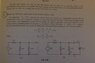# Nodal analysis with dependent voltage source

## Homework Statement

In attached image. Just curious as to how their nodal analysis is correct.

## Homework Equations

[/B]
##I_{in} = I_{out}##

## The Attempt at a Solution

Solution in image.

I am just not sure how they applied nodal analysis here to find that ##I_s = \frac{12}{12} + \frac{12}{6} + \frac{12-8}{4}##. If I'm not mistaken, they are just finding V/R, correct? But isn't the voltage in ALL the resistors dependent in some way on both the 12 V source attached and also the dependent voltage source? Why does it seem like they only included the dependent voltage only on the 4-ohm resistor? Is their solution correct?

#### Attachments

•Photo on 2015-12-12 at 9.44 PM.jpg
40 KB · Views: 407

Their solution is correct. If the applied independent source were changed to another value all the currents would be affected by the change, yet the resulting ratio of V/Is would turn out the same, yielding the same Rth.

Perhaps you have some doubt about how the node equation itself was written?

Their solution is correct. If the applied independent source were changed to another value all the currents would be affected by the change, yet the resulting ratio of V/Is would turn out the same, yielding the same Rth.

Perhaps you have some doubt about how the node equation itself was written?

I guess I'm just not entirely understanding why the dependent voltage source is not considered in these equations for the other 2 resistors. Doesn't this voltage source affect the true voltage across the 6- and 12-ohm resistor, thus requiring this to be included when analyzing the current passing through these resistors? It seems to me that they are considering the voltage through the 6- and 12-ohm resistor to be simply 12 V, but that doesn't appear obvious to me since there is more than one voltage source in this entire circuit.

I guess I'm just not entirely understanding why the dependent voltage source is not considered in these equations for the other 2 resistors. Doesn't this voltage source affect the true voltage across the 6- and 12-ohm resistor, thus requiring this to be included when analyzing the current passing through these resistors? It seems to me that they are considering the voltage through the 6- and 12-ohm resistor to be simply 12 V, but that doesn't appear obvious to me since there is more than one voltage source in this entire circuit.
The dependent source is not connected to the node where the 6 and 12 Ohm resistors connect; The external source is. So the external source must set their currents. Nothing can alter the potential difference imposed by a fixed voltage source and in this case the 12 V source is wired directly across those resistors.

•